Next: The Goal of the Up: Introduction Previous: Introduction   Index

# Remembrance of Things Past

You have been working with numbers for most of your life, and you know hundreds of facts about them. The first numbers you encountered were probably the positive integers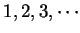. You learned that multiplication was repeated addition, and that, because 3 groups of apples, each of which contains 4 apples combine to give a group of 12 apples. Later you learned that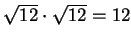. This did not mean that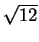groups of apples, each of which containedapples combine to give a group of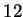apples. The definition had changed, but whatever it meant, you knew how to get the right answer''. At some point you met decimals. Since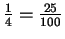, you knew that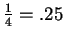.

However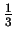was more of a problem. Although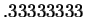was close to, the two numbers were not equal. Perhaps you considered infinite decimals, so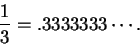Then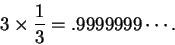But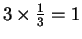, So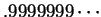must be equal to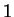. They don't look equal, but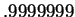was probably close enough. At first the fact that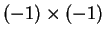was probably rather puzzling, but you got used to it after a while. You may have encountered the imaginary number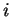such that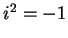and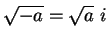whenis positive. Then you found thatandThis may have been unnerving. At some time numbers became identified with points on a line. Addition is straightforward: to add two numbers, you just slide the lines so that they share a common end, and combine them into one line.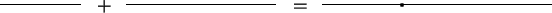but what does multiplication mean?Next: The Goal of the Up: Introduction Previous: Introduction   Index